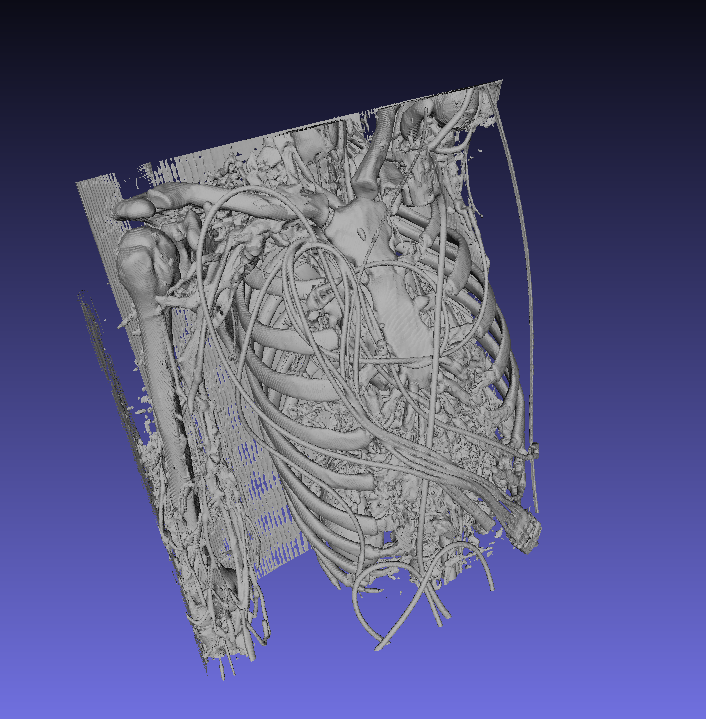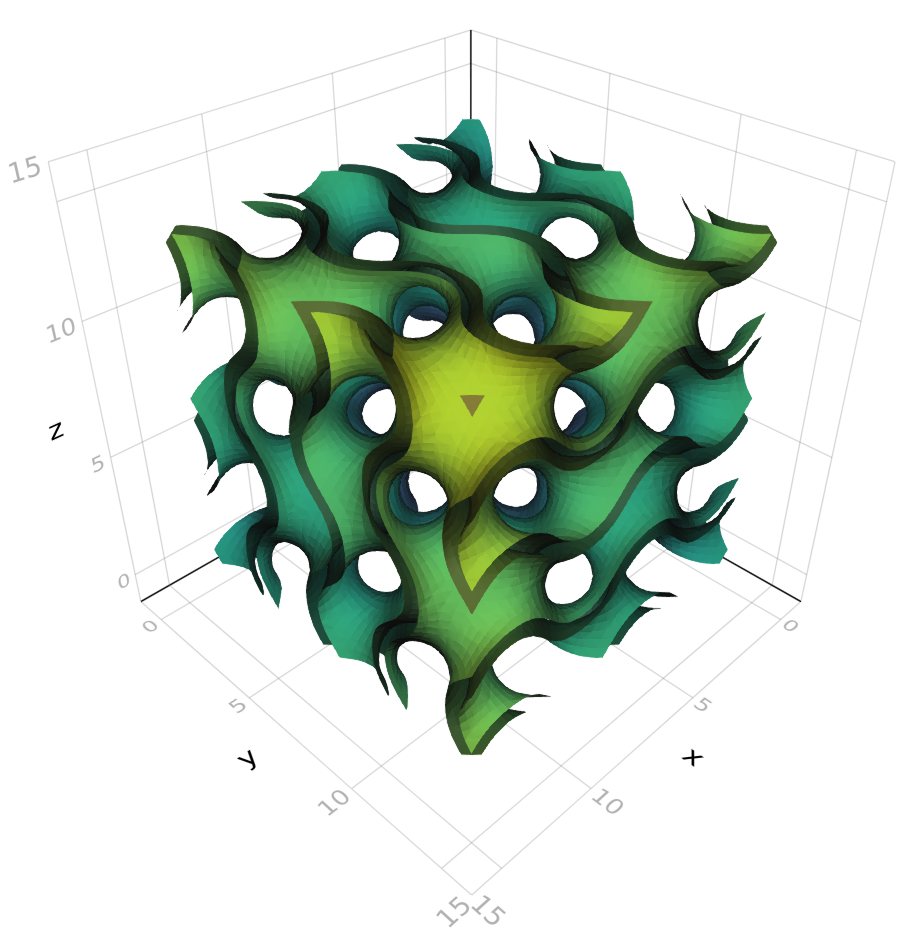Examples

# NRRD Data

The file for this example can be found here: http://www.slicer.org/slicerWiki/images/0/00/CTA-cardio.nrrd

``````
using FileIO
using NRRD
using Meshing
using MeshIO
using GeometryBasics

# load the file as an AxisArray

# use marching cubes with isolevel at 100
algo = MarchingCubes(iso=100, insidepositive=true)
# use marching tetrahedra with iso at 100
# algo = MarchingTetrahedra(iso=100, insidepositive=true)
# use Naive Surface Nets with iso at 100
# algo = NaiveSurfaceNets(iso=100, insidepositive=true)

# generate the mesh using marching cubes
mc = Mesh(ctacardio, algo)

# we can call isosurface to get a vector of points and vector of faces indexing to the points
# vertices, faces = isosurface(ctacardio, algo, Point{3,Float32}, TriangleFace{Int})

# save the file as a PLY file (change extension to save as STL, OBJ, OFF)
save("ctacardio_mc.ply", mc)``````# Functions

``````using Meshing
using FileIO # MeshIO should also be installed
using GeometryBasics

gyroid(v) = cos(v)*sin(v)+cos(v)*sin(v)+cos(v)*sin(v)
gyroid_shell(v) = max(gyroid(v)-0.4,-gyroid(v)-0.4)

# generate directly using GeometryBasics API
# Rect specifies the sampling intervals
gy_mesh = Mesh(gyroid_shell, Rect(Vec(0,0,0),Vec(pi*4,pi*4,pi*4)),
MarchingCubes(), samples=(50,50,50))

save("gyroid.ply", gy_mesh)

# view with Makie
import Makie
using LinearAlgebra
Makie.mesh(gy_mesh, color=[norm(v) for v in coordinates(gy_mesh)])``````